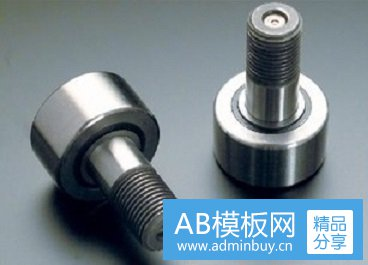# 滚轮滚针轴承局域本征区间的获取1.绝对值排序序列中位数

β,=)
1+1)2，i=1,2,,m(7-3)

i=1,2,,m(2+))
(7-4)式中A为绝对值排序序列中位数，i为时间阶段序号，N为第;个时间阶段获得的数据（data)个数，”为时间阶段数，)i)为绝对值排序序列中的第n个数据，为数据序号。滚轮轴承外圈采用外圈壁较厚的满装圆柱滚子轴承，滚轮的外径面有圆柱形和弧形，可根据使用场合设计来与滚道面配合。利用这种外圈，滚轮可以直接在滚道上滚动，并可以承受较重负荷和冲击负荷。
2.改进数据序列

y(b)<B;BSy(e)
m; y(b)为左序列首数据（data)。定义从小即大的职店0的排序列为左序列:左序列的数据个数为m; y(e)为右序列尾数据。定义从到大的顺序B.的排序序列为右序列，右序列的数据个数为

y,(n)=y(b)，i=,.,;-=-2.,N.即用y(b)代替y(n);如果(7-5)
y:(n)>y;(e)

y:(n)=y,(e)，i=1,-,m;n=1,2,,N 即用y(e)代替y(n)。

Z,(n,n)={z,(n;n,n)}，i=,2,,n-1-2,-,N。
(7-7)式中，Z(n,.n2)为改进数据（data)序列，z(n;n,n2)为改进数据序列的第n个数据，i 为时间阶段序号，n为数据序号，N为第i个时间阶段获得的数据个数，m为时间阶段数，n为左序列的数据个数，n2 为右序列的数据个数。复合滚轮轴承当中最主要的承载体，主要承受垂直方向的载荷和冲击负荷，具有很强的耐冲击性、耐磨性及抗腐蚀性。由于主滚轮为满装滚子轴承，亦可作为单向轴承单独使用。(7-6)
3.改进数据（data)序列平均值

i=1,2.,,m15
n.(n,n)=-Zz,(n;n,n),N-

D.= D(n,n2)=B

5.局城本征区间[Ki, Ka]

n=n2=1,2,,N2(7-10)

n=n2 =1,2,,N+12(7-11)且有(7-12)N

a=n+nN

Kn=y(b)，i=1,2,,mK2=y,(e)，i=1,2.,m于是，可以得到第i个时间阶段的局域本征区间
[K,K;]=[y;(b),y(e)]
(7-16)